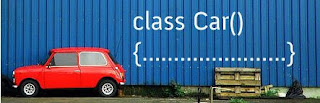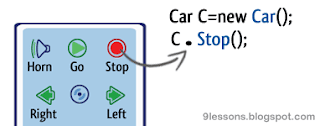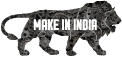What is an Object? - Easy Lesson
Tuesday, November 11, 2008

What is an Object? - Easy Lesson

Before the development of Object Oriented programming, the variables were considered as isolated entities. For example, suppose a program needs to handle data in car manufacturing company, here testing the cars having number, Color, Speed. Three separated variables will be declared for this.

int number,speed;
String color;These two statements do two things:
1)It says that the variable number and speed are integers and color is a string variable.
2)It also allocates storage for these variables.

If the program needs to keep track of various cars records. There will be more declarations that would be needed for each car, for example.

int number1,speed1;
String color1;

int number2,speed2;
String color2;

This approach can become very cumbersome.
The other drawback is that this approach completely ignores the fact that the three variables number,speed and color, are related and are a data associated with a single car.
In order to address these to drawbacks java use a class to create new types. For example to define a type that represents a car, we need storage space for two integers and a string. this is defined as follows:

class Car
{
int number;
int speed;
String color;
}

A variable can be declared as of type car as follows:

Car c,c1;

The car variables of this class(number,speed and color) can be accessed using the dot(.) operator.Creating an Object
The allocaton of memory of the reference type does not happen when the reference types are declared, as was the case of the primitive types, Simple diclaration of Non-primitive types does not allocate meomory space for the object.In fact, a variable that is declared with a class type is not the data itself, but it is a

reference to the data, The actual storage is allocated to the object as follows.

Car c;
c=new Car();

The first statement just allocates enough space for the reference. The second statement allocates the space, called an Object, for the two integers and a string. After these two statements are executed, the contents of the Car can be accessed through c.

Example using Object.

Let us now write a example that creates an object of the class Car and display the

number,speed, etc....

class Car
{
int number;
int speed;
String color;

void print_Number()
{
System.out.println("Car Number = " + number);
}

void print_Speed()
{
System.out.println("Car Speed = " + speed);
}

void Stop()
{
System.out.println("Car Stopped");
}

void Horn()
{
System.out.println("Hooooooo.......rn");
}

void Go()
{
System.out.println("Car Going");
}
}

public class Object_Car
{
public static void main(String args[])
{
Car c;
c=new Car();
c.number=401;
c.speed=90;
c.print_number();
c.print_speed();
c.Horn();
c.Stop();

Car c1;
c1=new Car();
c1.number=402;
c1.speed=80;
c1.print_number();
c1.print_speed();
c1.Go();
c1.Horn();

}
}

Output:
>javac Object_Car.java
>java Object_Car

Car Number = 401
Car Speed = 90
Car Stopped
Hooooooo.......rn

Car Number = 402
Car Speed = 80
Hooooooo.......rn
Car Going

1.ok,It is a nice explanation about Object.but it had a more similarities in explanationwith Head First Java.

2.The explanation talks about relating the three values as being the advancement done by OOP, is incorrect. Almost all programming languages had the concept(struct in C, Record in COBOL, PASCAL, etc). What was new in OOP was the introduciton of methods or functions as part of the class, This has not been highlighted, though your example does have methods in the class Car.
It would be better if you can make the change to talk about the methods in the initial explanation.

3.UR EXPLANATION IS NICE AND SIMPLE EXPLANATION FOR OBJECT.

4.very easy and so simple

5.very easy and simple